# The SURVEYFREQ Procedure

#### Risks and Risk Difference

The RISK option provides estimates of risks (binomial proportions) and risk differences fortables, together with their standard errors and confidence limits. Risk statistics include the row 1 risk, row 2 risk, overall risk, and risk difference. If you specify the RISK option, PROC SURVEYFREQ provides both column 1 and column 2 risks. You can request only column 1 (or only column 2) risks by specifying the RISK1 (or RISK2) option.

The column 1 risk for row 1 is the row 1 proportion for table cell (1,1). The column 1 risk estimate is computed as the ratio of the estimated total for table cell (1,1) to the estimated total for row 1,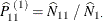where the total estimates are computed as described in the section Totals. The column 1 risk for row 2 is the row 2 proportion for table cell (2,1), which is estimated as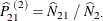The overall column 1 risk is the overall proportion in column 1, and its estimate is computed as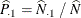The column 2 risk estimates are computed similarly.

The row 1 and row 2 risks are the same as the row proportions for atable, and their variances are computed as described in the section Row and Column Proportions. The overall risk is the overall proportion in the column, and its variance computation is described in the section Proportions. Confidence limits for the column 1 risk for row 1 are computed as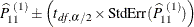where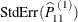is the standard error of the risk estimate, and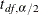is the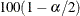percentile of the t distribution with df degrees of freedom calculated as described in the section Degrees of Freedom. The value of the confidence coefficientis determined by the ALPHA= option, which by default equals 0.05 and produces 95% confidence limits. Confidence limits for the other risks are computed similarly.

The risk difference is defined as the row 1 risk minus the row 2 risk. The estimate of the column 1 risk difference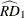is computed as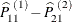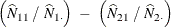The column 2 risk difference is computed similarly.

PROC SURVEYFREQ estimates the variance of the risk difference by using the variance estimation method that you request. If you request BRR variance estimation (VARMETHOD=BRR), the procedure estimates the variance as described in the section Balanced Repeated Replication (BRR). If you request jackknife variance estimation (VARMETHOD=JACKKNIFE), the procedure estimates the variance as described in the section The Jackknife Method.

If you do not specify the VARMETHOD= option or a REPWEIGHTS statement, the default variance estimation method is Taylor series (VARMETHOD=TAYLOR). By using Taylor series linearization, the variance estimate for the column 1 risk difference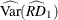can be expressed as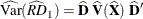where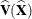is the covariance matrix of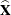,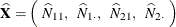and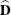is an array that contains the partial derivatives of the risk difference with respect to the elements of,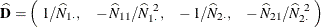See Wolter (1985, pp. 239–242) for details. The variance estimate for the column 2 risk difference is computed similarly.

The standard error of the column 1 risk difference is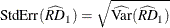Confidence limits for the column 1 risk difference are computed as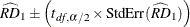whereis thepercentile of the t distribution with df degrees of freedom calculated as described in the section Degrees of Freedom. The value of the confidence coefficientis determined by the ALPHA= option, which by default equals 0.05 and produces 95% confidence limits. Confidence limits for the column 2 risk difference are computed in the same way.